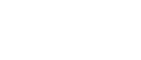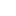#• PRODUCTS• SOLUTIONS• TUTORIALS• SUPPORT• ABOUT AKMGlobal | EnglishChoose your country or region
• Japan
• Global
• United States
• Europe
• China
• Korea
• Taiwan
• India

#• PRODUCTS
• SOLUTIONS
• TUTORIALS
• SUPPORT
• ABOUT AKM

# Combining AKM's current sensor with an A/D converter (2)

Episode 5. Continuing from the episode 4, introducing current sensing solutions by combining AKM's coreless current sensor and an ADC

#05 Basic Knowledge of ADCThis is a story that young A, who works in a fictional motor company deepens the knowledge of ADCs with a senior colleague K, and his boss, M, manager.

Young A

Datasheets for the current sensor and the ADC are separate, right? It's difficult to think about what all the characteristics will be!

Senior K

Let's talk about how to read basic characteristics in the datasheet this time. Which parameters are important to you for current detection?

Young A

First, current measurement range is important. Also, I care about output offset at zero current, current sensitivity, responsiveness, etc..

Senior K

Some parameters are determined only by the characteristics of the current sensor. Others depend on the combination with the ADC and peripheral circuits. Let's see what happens in case of the recommended circuit. (Figure 1)Figure 1. Circuit Diagram of the CQ3303 and the AK9235

Senior K

The current measurement range depends only on the specification of the current sensor. It is shown in the specification of, "Linear Sensing Range", and is ± 35A, for the CQ3303. (Figure 2)Figure 2. Connection Example of CQ3303 and AK9235

Young A

Apart from this, there is also, "maximum effective current"

Senior K

The, "maximum effective current", means the maximum current value that can be steadily passed through the current sensor. It is 20Arms in the case of the CQ3303. It is the point this value is an effective value.

Senior K

Next is the output offset at zero current. This also depends on the circuit configuration, but in the case of the recommended circuit, it can be confirmed by, "zero current voltage", of the current sensor and, "Offset Error", of the ADC. (Figure 3)Figure 3. Excerpt from the AK9235 Datasheet

Young A

Since VDD/2 (half of power supply voltage), is input to the AINN pin of the ADC, it is a differential input of 0V, is not it? ...... Well, but the specification of the current sensor is 2.5V. That's OK with VDD/2?

Senior K

This 2.5V is the value when VDD = 5V. Both the current sensitivity and the zero current voltage of the AKM current sensor are ratiometric. So even if it is written as 2.5V, the actual output is VDD/2.

Young A

Ratiometric? What?

Senior K

Ratiometric is the characteristic that the output fluctuates in proportion to supply voltage fluctuation.

Young A

Are the maximum and minimum offset values ± 20mV for the current sensor and ± 2mV for the ADC? You can ignore the ADC.

Senior K

Strictly, consider it as a sum of squares, let's convert it to the ADC output code with reference to the following description.

(Full Scale and LSB sizes are shown in the following equation)

Full Scale = 2 × VREF

1LSB = Full Scale / 4096 [V]

Young A

1 LSB is the voltage resolution per code, is it not? In the recommended circuit, since VDD/2 is input to the VREFP pin, it is 1.22 mV when VDD = 5V. Therefore, ± 20 mV is ± 16 LSB

Senior K

Good. Well then the current sensitivity. It can be calculated from, "current sensitivity", of current sensor and, "Full Scale Range" of the ADC.

Young A

..... Well, the unit changes between mV/A and V, which is hard to understand.

Senior K

It is good to calculate how much LSB the current variation in 1A will be at the ADC output.

Young A

Well, since the current sensitivity is 60mV/A, 60mV per 1A becomes the output voltage variation of the current sensor. When 60mV is divided by 1.22mV / LSB, it will be 49LSB.

Senior K

Yes. In the total of the current sensor and the ADC, the current sensitivity will be 49LSB/A. Strictly speaking, the, "Gain Error", of the ADC is also involved, but it is small enough to ignore. With this recommended circuit, 49LSB/A will not change even if the power supply fluctuates.

Young A

Is inputting VDD/2 to the VREFP pin and making the ADC ratiometric as well?

Senior K

You are right. For example, when VDD = 5.5 V, the current sensitivity of the current sensor becomes 66mV/A which is an increase of 10%. On the other hand, since the Full Scale Range of the ADC has also increased by 10%, 1 LSB is 1.34mV, (= 5.5 / 2 ^ 12). When dividing, it will be 49 LSB/A.

Young A

I'm now starting to get the image of how this works.

Senior K

Finally, responsiveness. The, "frequency characteristic", of the current sensor and, "Full Power Bandwidth", of the ADC, and what is important is the RC filter time constant on the board.

Young A

The current sensor has 1000kHz, and the ADC has 25MHz. The lower value should be considered and it's 1MHz, correct?

Senior K

The RC filter time constant is equal to the RC filter between the output of the current sensor and the input of the ADC. For 4.7Ω and 1nF, the cutoff frequency is 33.8MHz.

Young A

Well, after all, it means 1MHz.

Senior K

It is high enough now, but if you want to reduce the noise of the current sensor, you will narrow down the band further. If the cutoff frequency falls below 1MHz, this RC will limit the responsiveness.